4WX Internet Search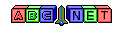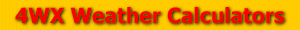# Vapor Pressure

From the user, an air temperature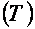and a dewpoint temperature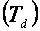are given.

To convert the saturated vapor pressure and/or the actual vapor pressure, the temperature values must be converted to degrees Celsius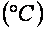For information on how to do this see the link below:

Then, the saturated vapor pressure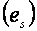and the actual vapor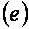can be calculated using the formula listed below: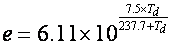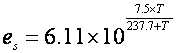For a bonus answer, after calculating the vapor pressures the relative humidity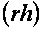can be calculated using the formula below:The vapor pressure answers will be in units of millibars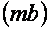or hectoPascals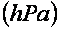To convert the vapor pressure to other units, see the link below:

pressureConversion.php

Back to 4WX Weather Calculators index page

4WX Calculators Credit and disclaimer